### Showing videos fromEddie Woo with a total of 4,051 videos06:28
Functions of Displacement (1 of 3: Basic Simple Harmonic Motion)
1y0m ago07:43
SHM - Other Centres of Motion (2 of 2: Determining attributes from the equation)
1y0m ago09:54
SHM - Other Centres of Motion (1 of 2: Rearranging with trigonometric identities)
1y0m ago09:03
Simple Harmonic Motion Example Question (3 of 3: Using graph symmetry)
1y0m ago09:25
Simple Harmonic Motion Example Question (2 of 3: Solving for time)
1y0m ago07:38
Simple Harmonic Motion Example Question (1 of 3: Determining period of motion)
1y0m ago10:38
Graphing Trigonometric Functions (Example: y = 3cos(x) - 2)
1y0m ago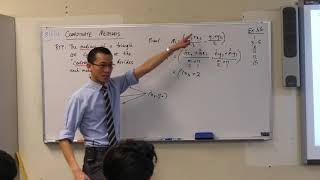08:09
Concurrence of Medians (2 of 2: Proof via coordinate geometry)
1y0m ago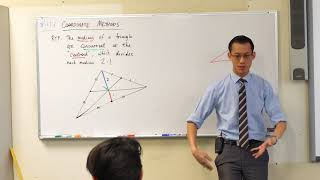08:09
Concurrence of Medians (1 of 2: Proof via similar triangles)
1y0m ago08:24
Intro to Straight Line Motion (3 of 3: Interpreting the equations)
1y0m ago07:10
Intro to Straight Line Motion (2 of 3: Unpacking a basic question)
1y0m ago08:57
Intro to Straight Line Motion (1 of 3: Overview of language)
1y0m ago08:43
Growth & Decay - Trout/Carp Problem (2 of 2: Drawing conclusions)
1y0m ago08:01
Growth & Decay - Trout/Carp Problem (1 of 2: Establishing the model)
1y0m ago07:12
Growth & Decay - Saltwater/Freshwater Problem (3 of 3: Interpreting the situation)
1y0m ago10:09
Growth & Decay - Saltwater/Freshwater Problem (2 of 3: Applying calculus)
1y0m ago09:51
Growth & Decay - Saltwater/Freshwater Problem (1 of 3: Understanding the constants)
1y0m ago09:18
Growth/Decay with Environmental Factors (2 of 2: Example question)
1y0m ago09:48
Growth/Decay with Environmental Factors (1 of 2: Difference in equations)
1y0m ago04:12
Exponential Growth Rates (1 of 2: Instantaneous)
1y0m ago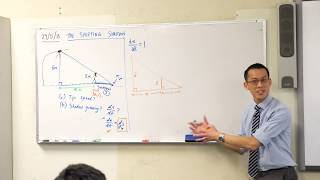07:54
Related Rates of Change - Shifting Shadow (2 of 2: Manipulating the derivatives)
1y0m ago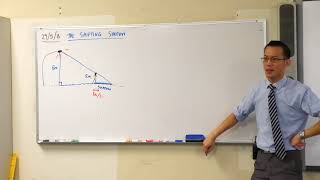06:57
Related Rates of Change - Shifting Shadow (1 of 2: Understanding the problem)
1y0m ago08:34
Related Rates of Change - Slipping Ladder (2 of 2: Working the equations)
1y0m ago08:35
Related Rates of Change - Slipping Ladder (1 of 2: Establishing the scenario)
1y0m ago12:22
Related Rates of a Balloon (3 of 3: Evaluating a rate of change)
1y1m ago07:14
Related Rates of a Balloon (2 of 3: Introducing the derivatives)
1y1m ago06:14
Related Rates of a Balloon (1 of 3: Describing the situation)
1y1m ago04:33
Graphs of Inverse Trigonometric Functions (2 of 2: Considering shifts through domain)
1y1m ago07:53
Graphs of Inverse Trigonometric Functions (1 of 2: Thinking through domain & range)
1y1m ago10:15
Combining Trigonometric & Inverse Trigonometric Functions
1y1m ago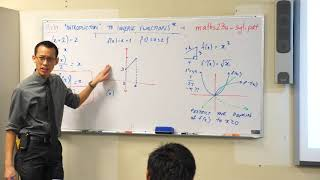06:33
Intro to Inverse Functions (4 of 4: Worked example)
1y1m ago07:01
Intro to Inverse Functions (3 of 4: Restricted domains)
1y1m ago08:47
Intro to Inverse Functions (2 of 4: Unifying geometric definition)
1y1m ago09:58
Intro to Inverse Functions (1 of 4: Overview & basic examples)
1y1m ago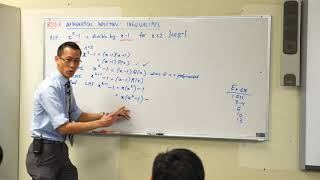06:26
Mathematical Induction: Polynomial Divisibility Proof
1y1m ago09:07
Mathematical Induction - Inequalities (4 of 4: Manipulating the inductive hypothesis)
1y1m ago05:30
Mathematical Induction - Inequalities (3 of 4: Starting with the assumption)
1y1m ago12:10
Mathematical Induction - Inequalities (2 of 4: Rearranging the k+1 case)
1y1m ago04:52
Mathematical Induction - Inequalities (1 of 4: General pointers)
1y1m ago07:04
Mathematical Induction Proof - Odd Integers (2 of 2: Proving the k+1 case)
1y1m ago07:00
Mathematical Induction Proof - Odd Integers (1 of 2: How to exclude even integers)
1y1m ago08:35
Mathematical Induction - Proving Divisibility by 4 (2 of 2: How to use the assumption)
1y1m ago11:58
Mathematical Induction - Proving Divisibility by 4 (1 of 2: Test and assumption)
1y1m ago09:05
Applications of Trigonometric Integrals (2 of 2: Introductory example)
1y1m ago05:16
Applications of Trigonometric Integrals (1 of 2: Fundamental properties)
1y1m ago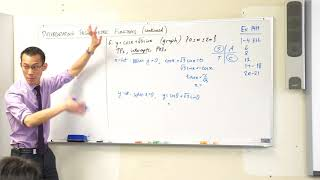07:58
Using Derivatives of Trigonometric Functions (2 of 2: Sketching a curve)
1y1m ago07:13
Using Derivatives of Trigonometric Functions (1 of 2: Finding a tangent)
1y1m ago07:43
Differentiating Trigonometric Functions (2 of 2: Example using quotient rule)
1y1m ago07:42
Differentiating Trigonometric Functions (1 of 2: Key results & chain rule)
1y1m ago08:34
Calculus of Trigonometric Functions (3 of 3: Proving the basic derivative)
1y1m ago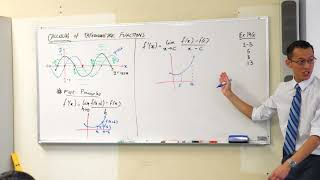06:36
Calculus of Trigonometric Functions (2 of 3: An alternative version of first principles)
1y1m ago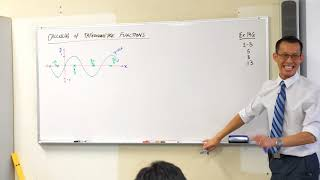07:38
Calculus of Trigonometric Functions (1 of 3: Using visual intuition)
1y1m ago09:05
Interpreting a Graph w/ Calculus (2 of 2: Evaluating an area)
1y1m ago07:32
Interpreting a Graph w/ Calculus (1 of 2: Sketching the curve)
1y2m ago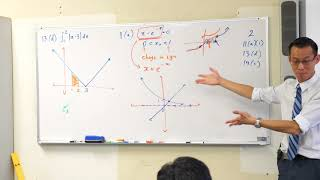03:16
Evaluating Definite Integral with Absolute Value
1y2m ago06:54
Proving existence of x-intercept in given domain (Bolzano's Theorem)
1y2m ago04:29
Solving Absolute Value Equation Example 3x + 2 = |2x - 1|
1y2m ago08:19
Behaviour of sinx & tanx for small x (3 of 3: Establishing the limit)
1y2m ago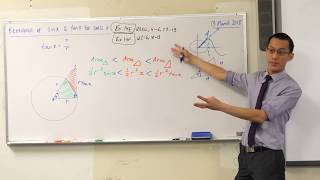08:20
Behaviour of sinx & tanx for small x (2 of 3: Geometric proof)
1y2m ago09:12
Behaviour of sinx & tanx for small x (1 of 3: Visualising on the unit circle)
1y2m ago11:07
Angle Between Two Lines (2 of 2: Dealing with vertical lines)
1y2m ago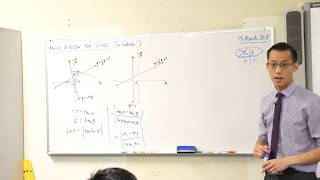09:01
Angle Between Two Lines (1 of 2: Calculating with radians)
1y2m ago09:08
Introduction to Trigonometric Expansions (3 of 3: Using them to prove identities)
1y2m ago